# Test: Electromechanical Indicating Instruments to Electrical & Electronics Measurements

## 10 Questions MCQ Test GATE Electrical Engineering (EE) 2023 Mock Test Series | Test: Electromechanical Indicating Instruments to Electrical & Electronics Measurements

Description
Attempt Test: Electromechanical Indicating Instruments to Electrical & Electronics Measurements | 10 questions in 30 minutes | Mock test for Electrical Engineering (EE) preparation | Free important questions MCQ to study GATE Electrical Engineering (EE) 2023 Mock Test Series for Electrical Engineering (EE) Exam | Download free PDF with solutions
QUESTION: 1

### A moving coil instrument has the following data: Number of turns = 100 Width of the coil = 20 mm Depth of the coil = 30 mm Flux density in the gap = 0.1 Wb/m2 If the control spring constant is 2 x 10-6 N-m/ degree, then the deflection of the pointer when the coil carries a current of 10 mA is

Solution:

The deflecting torque,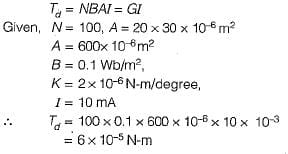At equilibrium,
Tc = Td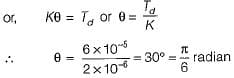Thus, the deflection of the pointer  = π/6 radian.

QUESTION: 2

### A PMMC uses a :

Solution:

A PMMC uses a moving coil and the magnet is permanent.

QUESTION: 3

### A moving iron movement uses

Solution:
QUESTION: 4

A 1 mA meter movement with an internal resistance of 100 Ω is to be converted into a 0-100 mA. The value of shunt resistant required is

Solution: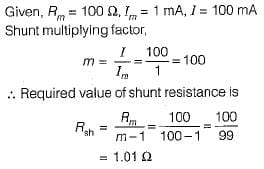QUESTION: 5

Which of the following is not a desired characteristic of shunts used in PMMC instruments?

Solution:

Shunts used in PMMC instruments are made up of manganin or constantan since they have very low temperature coefficient of resistance.

QUESTION: 6

To select the range, a multirange ammeter uses a

Solution:
QUESTION: 7

A D’Arsonval movement is

Solution:
QUESTION: 8

What is the value of multiplier resistance on the 50 V range of a dc voltmeter that uses a 500 μA meter movement with an internal resistance of 1 kΩ?

Solution:

The sensitivity of 500 μA meter movement is given by,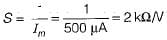The value of the multiplier resistance can be obtained as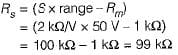QUESTION: 9

Solution:

Higher the sensitivity of the voltmeter, lower the loading effect and vice-versa.

QUESTION: 10

Assertion (A): Gravity control is not suited for indicating instruments in general and portable instruments in particular.
Reason (R): Gravity control is used in only vertically mounted instruments.

Solution:

Gravity control is not suited for indicating instruments in general and portable instruments in particular because for using this type of control, the instruments must be mounted in level position otherwise there will be a very serious zero error. Gravity control is used in only vertically mounted instruments. Hence, reason is also a true statement but, not a correct explanation of assertion.Use Code STAYHOME200 and get INR 200 additional OFF Use Coupon Code